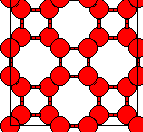Crystal Lattice Structures: Creation Date: 25 Mar 2002 Last Modified: 21 Oct 2004

# Tetrahedrally Bonded Carbon with Four Member RingsYou can now

• Prototype: C (hypothetical 4-member ring structure)
• Pearson Symbol: tI8
• Space Group: I4/mmm (Cartesian and lattice coordinate listings available)
• Number: 139
• Reference: P. Schultz et al., Phys. Rev. B 59, 733-741 (1999)
• Primitive Vectors:  A1 = a X A2 = a Y A3 = ½ a X + ½ a Y + ½ c Z
• Basis Vectors  B1 = + x A1 + x A2 = + x a X + x a Y (C) (8h) B2 = - x A1 + x A2 = - x a X + x a Y (C) (8h) B3 = - x A1 - x A2 = - x a X - x a Y (C) (8h) B4 = + x A1 - x A2 = + x a X - x a Y (C) (8h)

### See these vectors LaTeX output format.

Go back to the Carbon Structures page.

Go back to Crystal Lattice Structure page.

 Structures indexed by: This is a mirror of an old page created at theNaval Research LaboratoryCenter for Computational Materials ScienceThe maintained successor is hosted at http://www.aflowlib.org/CrystalDatabase/ and published as M. Mehl et al., Comput. Mater. Sci. 136 (Supp.), S1-S828 (2017).